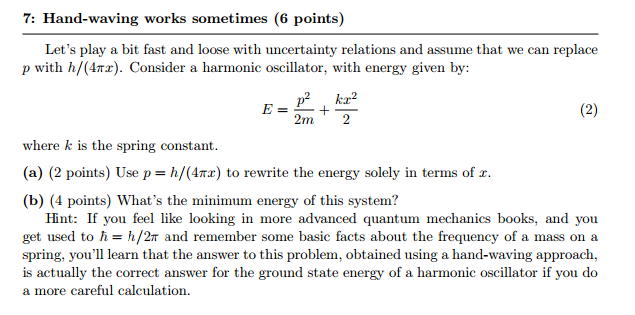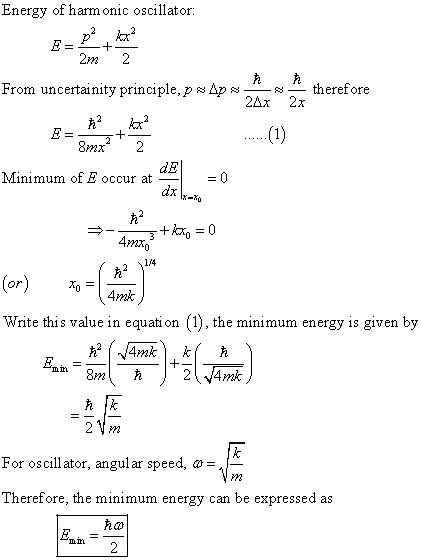# part b only 7: Hand-waving works sometimes (6 points Let's play a bit fast and loose...

part b only7: Hand-waving works sometimes (6 points Let's play a bit fast and loose with uncertainty relations and assume that we can replace p with h/(47Tr). Consider a harmonic oscillator, with energy given by p2 kar 2 (2) 2m. 2 where k is the spring constant. (a) (2 points) Use p h/(4Tr) to rewrite the energy solely in terms of ar (b) (4 points) What's the minimum energy of this system? Hint: If you feel like looking in more advanced quantum mechanics books, and you get used to h h/2T and remember some basic facts about the frequency of a mass on a spring, you'll learn that the answer to this problem, obtained using a hand-waving approach is actually the correct answer for the ground state energy of a harmonic oscillator if you do a more careful calculation.#### Earn Coin

Coins can be redeemed for fabulous gifts.